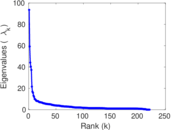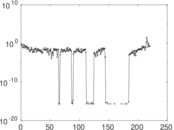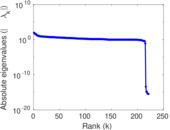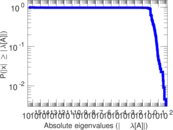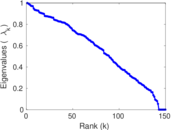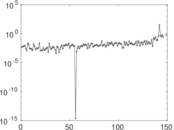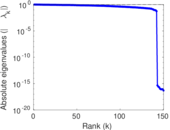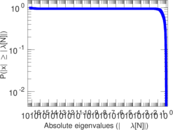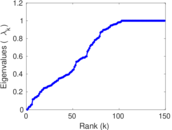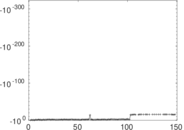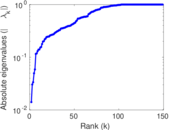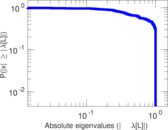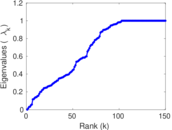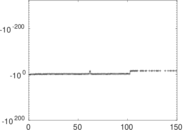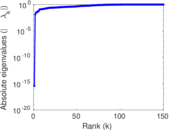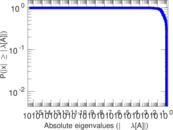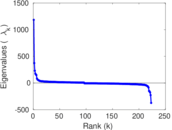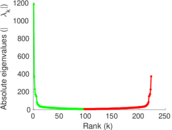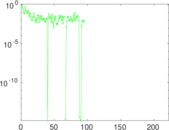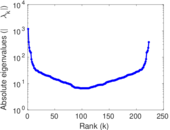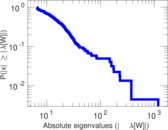# Wikiquote edits (sq)

This is the bipartite edit network of the Albanian Wikiquote. It contains users and pages from the Albanian Wikiquote, connected by edit events. Each edge represents an edit. The dataset includes the timestamp of each edit.

 Code `qsq` Internal name `edit-sqwikiquote` Name Wikiquote edits (sq) Data source http://dumps.wikimedia.org/ AvailabilityDataset is available for download Consistency checkDataset passed all tests Category Authorship network Dataset timestamp 2017-10-20 Node meaning User, article Edge meaning Edit Network formatBipartite, undirected Edge typeUnweighted, multiple edges Temporal dataEdges are annotated with timestamps

## Statistics

 Size n = 1,200 Left size n1 = 223 Right size n2 = 977 Volume m = 3,128 Unique edge count m̿ = 1,829 Wedge count s = 62,240 Claw count z = 2,707,293 Cross count x = 110,395,485 Square count q = 14,123 4-Tour count T4 = 366,854 Maximum degree dmax = 398 Maximum left degree d1max = 398 Maximum right degree d2max = 126 Average degree d = 5.213 33 Average left degree d1 = 14.026 9 Average right degree d2 = 3.201 64 Fill p = 0.008 394 88 Average edge multiplicity m̃ = 1.710 22 Size of LCC N = 971 Diameter δ = 14 50-Percentile effective diameter δ0.5 = 3.769 99 90-Percentile effective diameter δ0.9 = 5.891 95 Median distance δM = 4 Mean distance δm = 4.481 28 Gini coefficient G = 0.734 396 Balanced inequality ratio P = 0.198 529 Left balanced inequality ratio P1 = 0.169 118 Right balanced inequality ratio P2 = 0.266 944 Relative edge distribution entropy Her = 0.849 736 Power law exponent γ = 3.052 36 Tail power law exponent γt = 2.101 00 Tail power law exponent with p γ3 = 2.101 00 p-value p = 0.062 000 0 Left tail power law exponent with p γ3,1 = 1.681 00 Left p-value p1 = 0.047 000 0 Right tail power law exponent with p γ3,2 = 2.311 00 Right p-value p2 = 0.064 000 0 Degree assortativity ρ = −0.248 740 Degree assortativity p-value pρ = 3.414 49 × 10−27 Spectral norm α = 93.518 4 Algebraic connectivity a = 0.014 070 5 Spectral separation |λ1[A] / λ2[A]| = 1.580 37 Controllability C = 746 Relative controllability Cr = 0.634 354

## Plots

### Fruchterman–Reingold graph drawing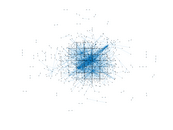### Degree distribution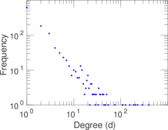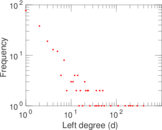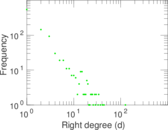### Cumulative degree distribution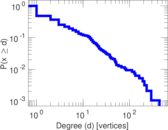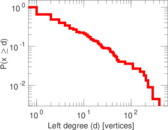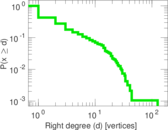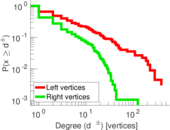### Lorenz curve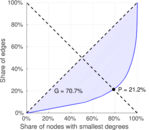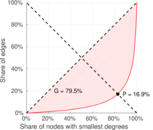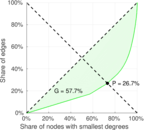### Spectral distribution of the adjacency matrix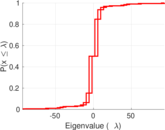### Spectral distribution of the normalized adjacency matrix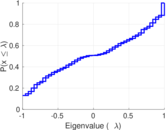### Spectral distribution of the Laplacian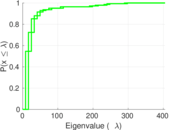### Spectral graph drawing based on the adjacency matrix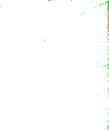### Spectral graph drawing based on the Laplacian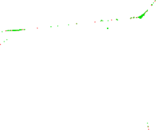### Spectral graph drawing based on the normalized adjacency matrix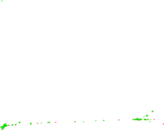### Degree assortativity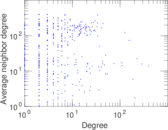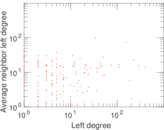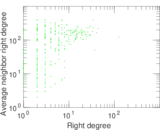### Zipf plot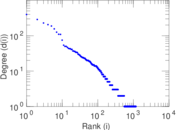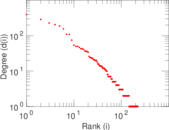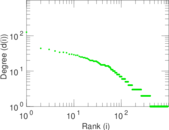### Hop distribution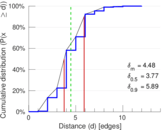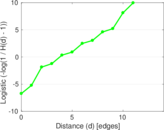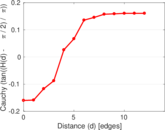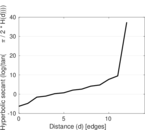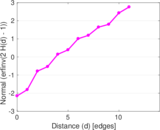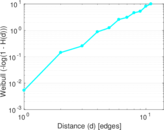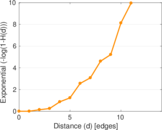### Double Laplacian graph drawing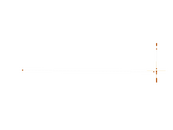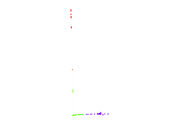### Delaunay graph drawing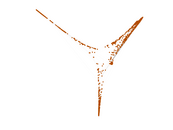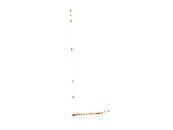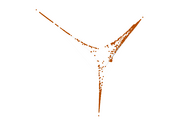### Edge weight/multiplicity distribution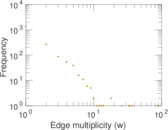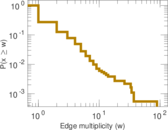### Temporal distribution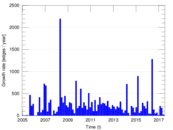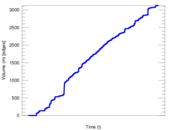### Temporal hop distribution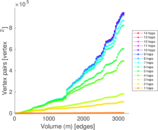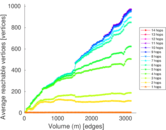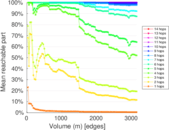### Diameter/density evolution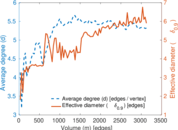### Matrix decompositions plots